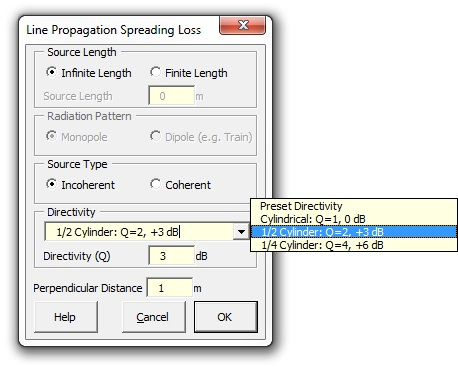### Strutt Help

Strutt|Propagation|Line Source provides the correction for cylindrical sound radiation from a line source, given the perpendicular distance from the source (d) in metres.Distance correction can be calculated for either finite or infinite line sources and coherent sources (e.g. tonal noise source) or incoherent sources (broadband noise source e.g. traffic, pipe, conveyor belt).

Infinite Line Source calculates the sound pressure level (SPL) for the following:

Incoherent Line Source is calculated using

L_p = L prime _W - 6 - 10log_10 d

Coherent Line Source is calculated using

L_p = L prime _W - 8 - 10log_10 d

Finite Line Source calculates the SPL for the following:

Incoherent Line Source is calculated using

L_p = L prime _W - 8 - 10log_10 d + 10log_10 [2tan^(-1)(L/(2d))]

Where L is source length and d is perpindicular distance to receiver.

A Coherent Line Source of finite length has the same SPL as a finite length incoherent source, for large distances from the source compared with the source length (d > L//2).

Close to a coherent source of finite length (d < L//10), the SPL normal to the centre of the source will be the same as for an infinite coherent source.

For distances in between, the SPL for coherent finite length sources is interpolated.

N.B. the sound pressure level L_p is calculated using the sound power per unit length L prime _W  (dB//m), not the overall sound power L_W (dB).

It should be noted that the Finite Line Source calculation effectively includes an angle of view correction (the 10log_10[2tan^(-1)(L//2d)] term), while Infinite Line Source does not. If infinite line source is used, a separate Angle of View Correction should be applied if necessary.

The Finite Line Source calculation also assumes that the midpoint of the source is aligned with the receiver location - i.e. that the source is symmetrical around the receiver. If this is not the case, use the infinite line source calculation and the Angle of View correction.

To calculate sound pressure level where the spacing between sources is finite, use the Array of Point Sources calculation.

References:

• Transportation Noise Reference Book p2/8
• Bies, D., Hansen, C., Engineering Noise Control, 3rd Edition, pp188-192